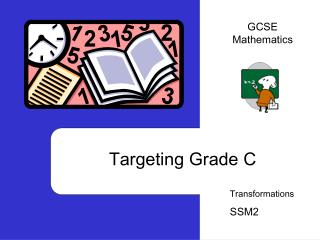DownloadDownload PresentationTélécharger la présentation- - - - - - - - - - - - - - - - - - - - - - - - - - - E N D - - - - - - - - - - - - - - - - - - - - - - - - - - -
##### Presentation Transcript

1. GCSE Mathematics Targeting Grade C Transformations SSM2

2. C C The big picture

3. Can you: • Reflect a 2D shape in a line and state the equation of the line. • Rotate a 2D shape about a point and state the angle, direction and centre of rotation. • Try a question • Translate a 2D shape and describe the tranlation • Enlarge 2D shapes using positive scale factors • Describe a single transformation that is equivalent to a combination of transformations • Try a test Practice 1:Reflection Practice 2: Rotation Try a question Practice 3: Translation Practice 4: Enlargement Practice 5: Combinations of transformations Exam questions If not you need

4. Practice 1 T/ T Reflect Triangle ‘T’ about the dotted line Are you ready for the answer? Remember reflection does not change shape or length!

5. Practice 1 S Are you ready for the answers? Reflect shape ‘S’ about the dotted line What happens when you reflect in a diagonal line? Hint look at the angles on the diagonal lines for corresponding points.

6. Practice 2 T Rotate Triangle ‘T’ 90º Anticlockwise about the centre Remember to ask for tracing paper in the exam! Are you ready for the answer?

7. Practice 2 T Remember-3 things! centre of rotation, direction of rotation and angle of rotation Rotate Triangle ‘T’ 180º anticlockwise, about the centre Are you ready for the answer?

8. Y axis Try a question Reflection and rotation only change position. 1. Draw the line of reflection for A to B. 2. State the equation of the line of reflection. 3. Rotate A, centre of rotation (0,0), 90º anticlockwise. Label C 8 7 6 5 4 3 2 1 -1 -2 -3 -4 -5 -6 -7 A C X axis -7 -6 -5 -4 -3 -2 -1 1 2 3 4 5 6 7 8 All y values are –1, so equation of line is y = -1 B Are you ready for the answers?

9. Practice 3 8 7 6 5 4 3 2 1 -1 -2 -3 -4 -5 -6 -7 Translate A, 4 units left and 2 units up. Translate B A/ A -7 -6 -5 -4 -3 -2 -1 1 2 3 4 5 6 7 8 Translation: Same shape, same size, same orientation B/ B Are you ready for the answers?

10. Enlargement Practice 4 B Are you ready for the answer? Enlarge B Scale Factor 2 But where should it go on the grid???? What happens to lengths of sides? What happens to shape?

11. Practice 4 1. Work out the scale factors of each enlargement from A. 2. Start with D, work out each scale factor enlargement. 3. Shape E cannot be seen. From E shape D is a scale factor enlargement of ¼. Describe shape E. D C B A 3. 2. Shape E is the same shape as D, but the corresponding sides are 4 times longer. 1. A to B is SF ½ A to C is SF 3 A to D is SF 2 D to A is SF ½ D to B is SF ½ D to C is SF 1 ½ Are you ready for the answers?

12. Y axis 5 Practice 4 G X axis 5 10 Use the radial lines from the centre of enlargement to position the enlargement. What happens to shape? Sides? Enlarge G Scale Factor ½ Centre of enlargement the origin, (0,0) Are you ready for the answer?

13. (a) (i) Describe fully the single transformation that takes the shaded triangle to triangle A. (2) ............................................................................................................... (ii) On the grid above translate the shaded triangle by 2 squares to the right and 4 squares down. (1) Practice 5 Are you ready for the answers? • (i)A reflection in the line x = -1 • (ii)Purple triangle

14. (b) Triangle P is an enlargement of the shaded triangle. (i) What is the scale factor of the enlargement? Answer .........................................................…(1) (ii) What is the centre of enlargement? Answer (..........................., .............................) (1) Are you ready for the answers? b(i) ½ (ii) (-2,-1)

15. Try a question Hint Read the question carefully!! Answer fully Are you ready for the answer? Remember there are 2 parts to describe an enlargement. It is an enlargement, scale factor ½, centre of enlargement (0,3)

16. Exam question Are you ready for the answers? • A • E • Reflection in the line x = -2 • Write down the letter of the triangle • after the shaded triangle is reflected in the line x = 3 (1) • After the shaded triangle is translated by 3 squares to the right and 5 squares down. (1) • Describe fully the single transformation which takes triangle F on to triangle G • (2)